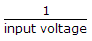# Electronics and Communication Engineering - Analog Electronics

46.

In an op-amp comparator, the positive or negative output is

 A. saturated B. amplifier C. unsaturated D. any of the above

Explanation:

No answer description available for this question. Let us discuss.

47.

In logarithmic amplifiers, output voltage is equal to

 A. K.log10 (input voltage) B. K.loge (input voltage) C. input voltage D.Explanation:

No answer description available for this question. Let us discuss.

48.

In a series regulated power supply circuit the voltage gain of pass transistor satisfies the condition

 A. Av → ∞ B. 1 << Av → ∞ C. Av ≈ 1 D. Av << 1

Explanation:

No answer description available for this question. Let us discuss.

49.

Occasionally a discrete amplifier user RF chokes instead of collector resistors. Such amplifiers are inherently

 A. low pass amplifiers B. high pass amplifiers C. band pass amplifiers D. none of the above

Explanation:

No answer description available for this question. Let us discuss.

50.

In oscillator circuits the energy feedback to its input terminal from output is

 A. always in phase with the input signal B. 90° out of phase with the input signal C. 180° out of phase with the input signal D. 270° out of phase with the input signal